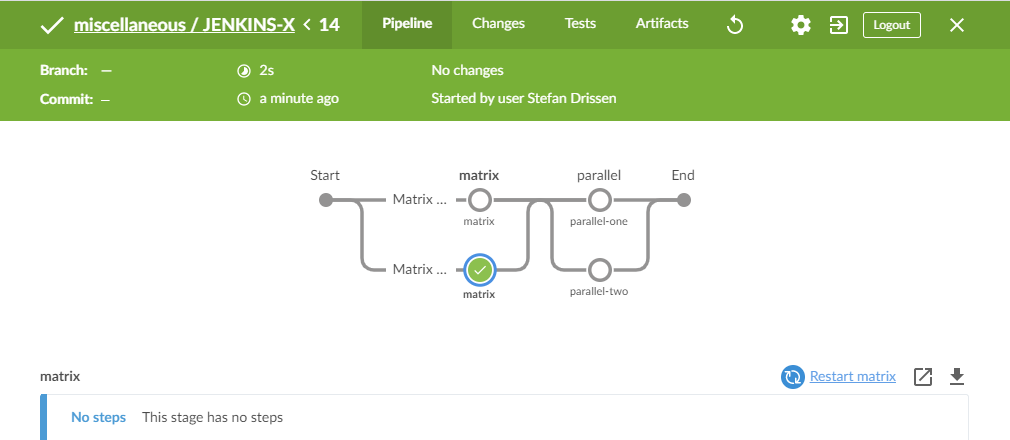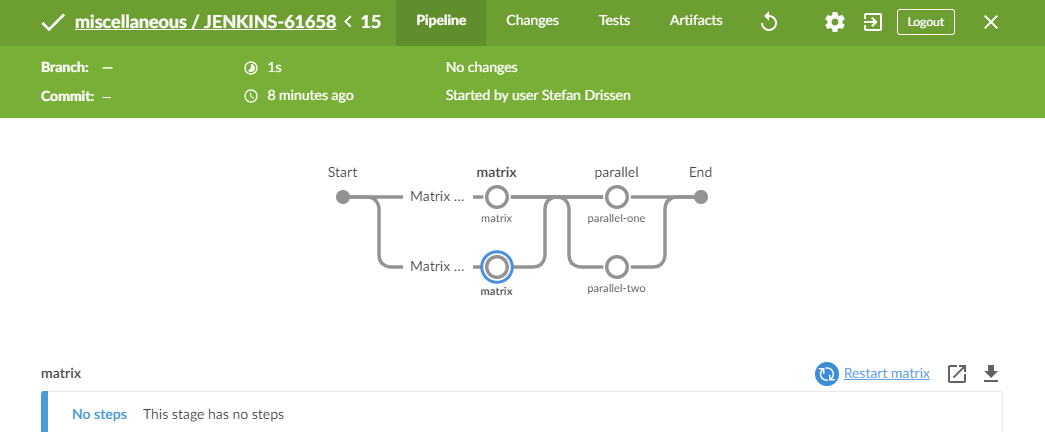# Blue Ocean Pipeline: only first skipped cell of matrix shown as skipped

XMLWordPrintable

#### Details

• Type:Bug
• Status: Open
• Priority:Major
• Resolution: Unresolved
• Component/s:
• Labels:
None
• Environment:
Jenkins 2.226
• Similar Issues:

#### Description

When stages within a matrix are skipped using the 'when' directive, the pipeline result is not rendered correctly. The first cell of the matrix is correctly shown as 'Skipped', however subsequent cells are shown as 'Passed' (in 0s). This is very confusing.

```#!groovy
pipeline {
agent none
parameters {
booleanParam( name: 'matrix', defaultValue: true )
booleanParam( name: 'parallel', defaultValue: true )
}
stages {

stage( 'matrix' ) {
matrix {
axes {
axis {
name 'server'
values 'server-one', 'server-two'
}
}
stages {
stage( 'matrix' ) {
agent {
label "\${server}"
}
when {
expression { params.matrix }
}
steps {
echo "matrix \${server}"
}
}
}
}
}

stage( 'parallel' ) {

parallel {

stage( 'parallel-one' ) {
agent {
label 'server-one'
}
when {
expression { params.parallel }
}
steps {
echo 'parallel-one'
}
}        stage( 'parallel-two' ) {
agent {
label 'server-two'
}
when {
expression { params.parallel }
}
steps {
echo 'parallel-two'
}
}

}
}

} // stages

} // pipeline
```#### Activity

Field Original Value New Value
Description When stages within a matrix are skipped using the when, directive, the build is not rendered correctly. The first cell of the matrix is correctly shown as 'Skipped', the subsequent cells are shown as 'Passed' (in 0s). This is very confusing.

{noformat}
#!groovy
pipeline {
agent none
parameters {
booleanParam( name: 'matrix', defaultValue: true )
booleanParam( name: 'parallel', defaultValue: true )
}
stages {

stage( 'matrix' ) {
matrix {
axes {
axis {
name 'server'
values 'server-one', 'server-two'
}
}
stages {
stage( 'matrix' ) {
agent {
label "\${server}"
}
when {
expression { params.matrix }
}
steps {
echo "matrix \${server}"
}
}
}
}
}

stage( 'parallel' ) {

parallel {

stage( 'parallel-one' ) {
agent {
label 'server-one'
}
when {
expression { params.parallel }
}
steps {
echo 'parallel-one'
}
} stage( 'parallel-two' ) {
agent {
label 'server-two'
}
when {
expression { params.parallel }
}
steps {
echo 'parallel-two'
}
}

}
}

} // stages

} // pipeline
{noformat}
!image-2020-03-24-09-29-52-211.png!

When stages within a matrix are skipped using the 'when' directive, the build result is not rendered correctly. The first cell of the matrix is correctly shown as 'Skipped', however subsequent cells are shown as 'Passed' (in 0s). This is very confusing.

{noformat}
#!groovy
pipeline {
agent none
parameters {
booleanParam( name: 'matrix', defaultValue: true )
booleanParam( name: 'parallel', defaultValue: true )
}
stages {

stage( 'matrix' ) {
matrix {
axes {
axis {
name 'server'
values 'server-one', 'server-two'
}
}
stages {
stage( 'matrix' ) {
agent {
label "\${server}"
}
when {
expression { params.matrix }
}
steps {
echo "matrix \${server}"
}
}
}
}
}

stage( 'parallel' ) {

parallel {

stage( 'parallel-one' ) {
agent {
label 'server-one'
}
when {
expression { params.parallel }
}
steps {
echo 'parallel-one'
}
} stage( 'parallel-two' ) {
agent {
label 'server-two'
}
when {
expression { params.parallel }
}
steps {
echo 'parallel-two'
}
}

}
}

} // stages

} // pipeline
{noformat}
!image-2020-03-24-09-29-52-211.png!

 Description When stages within a matrix are skipped using the 'when' directive, the build result is not rendered correctly. The first cell of the matrix is correctly shown as 'Skipped', however subsequent cells are shown as 'Passed' (in 0s). This is very confusing.   {noformat} #!groovy pipeline {   agent none   parameters {     booleanParam( name: 'matrix', defaultValue: true )     booleanParam( name: 'parallel', defaultValue: true )   }   stages {     stage( 'matrix' ) {       matrix {         axes {           axis {             name 'server'             values 'server-one', 'server-two'           }         }         stages {           stage( 'matrix' ) {             agent {               label "\${server}"             }             when {               expression { params.matrix }             }             steps {               echo "matrix \${server}"             }           }         }       }     }          stage( 'parallel' ) {            parallel {         stage( 'parallel-one' ) {           agent {             label 'server-one'           }           when {             expression { params.parallel }           }           steps {             echo 'parallel-one'           }         } stage( 'parallel-two' ) {           agent {             label 'server-two'           }           when {             expression { params.parallel }           }           steps {             echo 'parallel-two'           }         }                }     }        } // stages } // pipeline {noformat} !image-2020-03-24-09-29-52-211.png! When stages within a matrix are skipped using the 'when' directive, the pipeline result is not rendered correctly. The first cell of the matrix is correctly shown as 'Skipped', however subsequent cells are shown as 'Passed' (in 0s). This is very confusing.   {noformat} #!groovy pipeline {   agent none   parameters {     booleanParam( name: 'matrix', defaultValue: true )     booleanParam( name: 'parallel', defaultValue: true )   }   stages {     stage( 'matrix' ) {       matrix {         axes {           axis {             name 'server'             values 'server-one', 'server-two'           }         }         stages {           stage( 'matrix' ) {             agent {               label "\${server}"             }             when {               expression { params.matrix }             }             steps {               echo "matrix \${server}"             }           }         }       }     }          stage( 'parallel' ) {            parallel {         stage( 'parallel-one' ) {           agent {             label 'server-one'           }           when {             expression { params.parallel }           }           steps {             echo 'parallel-one'           }         } stage( 'parallel-two' ) {           agent {             label 'server-two'           }           when {             expression { params.parallel }           }           steps {             echo 'parallel-two'           }         }                }     }        } // stages } // pipeline {noformat} !image-2020-03-24-09-29-52-211.png!
 Attachment image-2020-03-25-17-31-20-694.png [ 50750 ]
 Attachment image-2020-03-25-17-31-24-907.png [ 50751 ]

I just discovered that if I use the beforeAgent option on the when directive:

```#!groovy

pipeline {

agent none

parameters {
booleanParam( name: 'matrix', defaultValue: true )
booleanParam( name: 'parallel', defaultValue: true )
}

stages {

stage( 'matrix' ) {

matrix {
axes {
axis {
name 'server'
values 'server-one', 'server-two'
}
}
stages {
stage( 'matrix' ) {
agent {
label "\${server}"
}
when {
beforeAgent true
expression { params.matrix }
}
steps {
echo "matrix \${server}"
}
}
}
}

}

stage( 'parallel' ) {

parallel {

stage( 'parallel-one' ) {
agent {
label 'server-one'
}
when {
expression { params.parallel }
}
steps {
echo 'parallel-one'
}
}
stage( 'parallel-two' ) {
agent {
label 'sever-two'
}
when {
expression { params.parallel }
}
steps {
echo 'parallel-two'
}
}

}

}

} // stages

} // pipeline

```

That the visual result is a lot better:There is still some confusion as to what is what, since the first matrix cell reports that it has been skipped, whereas the second cell reports that it was not built.

ShowStefan Drissen added a comment - I just discovered that if I use the beforeAgent option on the when  directive:     #!groovy pipeline { agent none parameters { booleanParam( name: 'matrix' , defaultValue: true ) booleanParam( name: 'parallel' , defaultValue: true ) } stages { stage( 'matrix' ) { matrix { axes { axis { name 'server' values 'server-one' , 'server-two' } } stages { stage( 'matrix' ) { agent { label "\${server}" } when { beforeAgent true expression { params.matrix } } steps { echo "matrix \${server}" } } } } } stage( 'parallel' ) { parallel { stage( 'parallel-one' ) { agent { label 'server-one' } when { expression { params.parallel } } steps { echo 'parallel-one' } } stage( 'parallel-two' ) { agent { label 'sever-two' } when { expression { params.parallel } } steps { echo 'parallel-two' } } } } } // stages } // pipeline That the visual result is a lot better: There is still some confusion as to what is what, since the first matrix cell reports that it has been skipped , whereas the second cell reports that it was  not   built .

#### People

Assignee:Unassigned
Reporter:Stefan Drissen